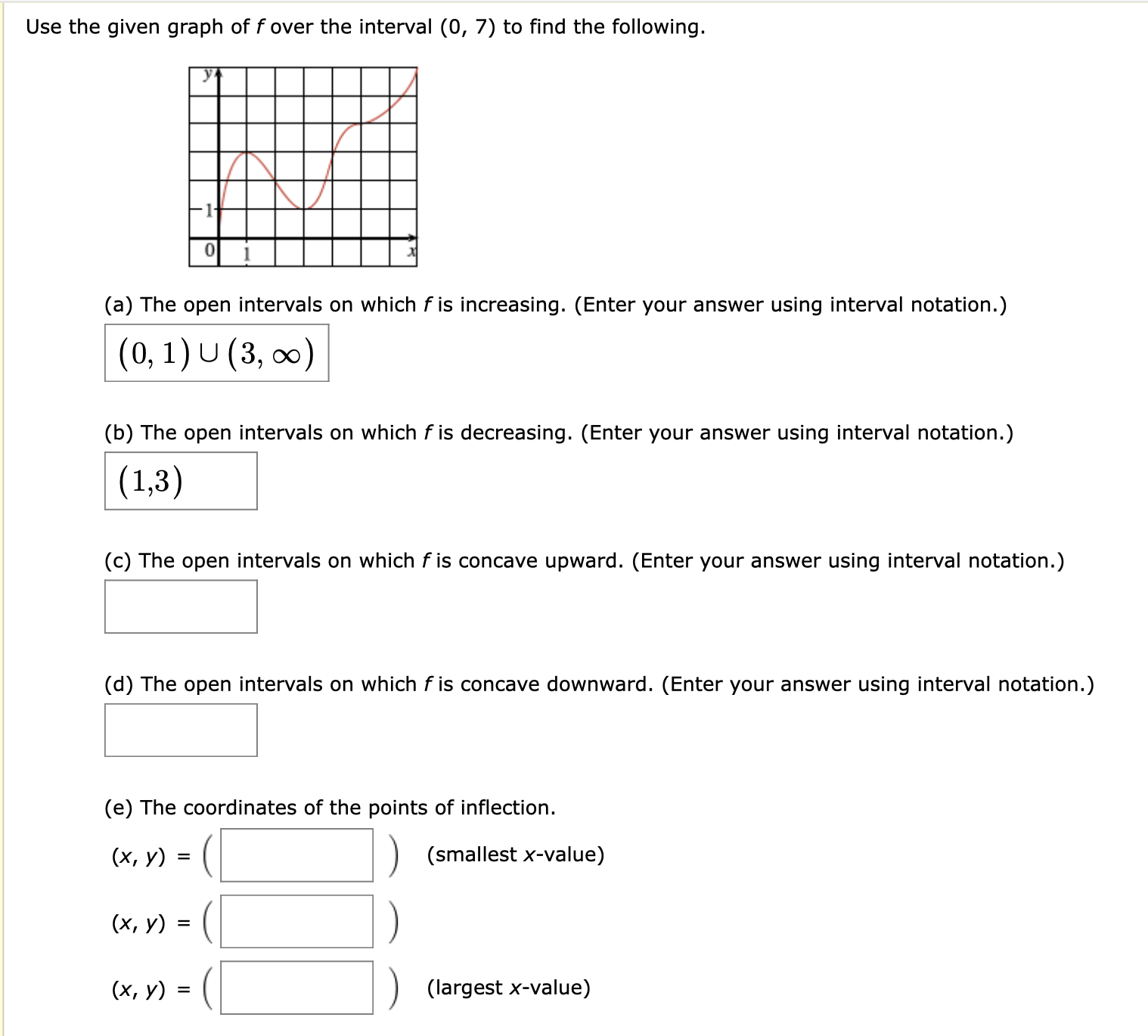# Use the given graph of fover the interval (0, 7) to find the following. (a) The open intervals on which f is increasing. (Enter your answer using interval notation.) (0, 1)U (3, oo) (b) The open intervals on which f is decreasing. (Enter your answer using interval notation.) (1,3) (c) The open intervals on which f is concave upward. (Enter your answer using interval notation.) (d) The open intervals on which f is concave downward. (Enter your answer using interval notation.) (e) The coordinates of the points of inflection (х, у) (smallest x-value) (х, у) %3 (х, у) %3 (largest x-value)

Questionhelp_outlineImage TranscriptioncloseUse the given graph of fover the interval (0, 7) to find the following. (a) The open intervals on which f is increasing. (Enter your answer using interval notation.) (0, 1)U (3, oo) (b) The open intervals on which f is decreasing. (Enter your answer using interval notation.) (1,3) (c) The open intervals on which f is concave upward. (Enter your answer using interval notation.) (d) The open intervals on which f is concave downward. (Enter your answer using interval notation.) (e) The coordinates of the points of inflection (х, у) (smallest x-value) (х, у) %3 (х, у) %3 (largest x-value) fullscreen

1 Rating

### Want to see this answer and more?

Experts are waiting 24/7 to provide step-by-step solutions in as fast as 30 minutes!*

*Response times vary by subject and question complexity. Median response time is 34 minutes and may be longer for new subjects.
Tagged in
MathCalculus

### Other